# Denumerant

(diff) ← Older revision | Latest revision (diff) | Newer revision → (diff)

The number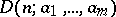of partitions of an integerinto parts equal to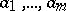, i.e. the number of solutions in non-negative integers of the equation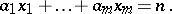The generating function of the denumerants is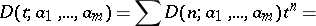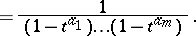The simplest method of computing a denumerant is by Euler's recurrence relation:Explicit formulas for certain denumerants may be obtained from the following theorem: Ifis the least common multiple of the numbers, then the denumerant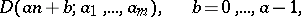is a polynomial of degreewith respect to.

How to Cite This Entry:
Denumerant. Encyclopedia of Mathematics. URL: http://encyclopediaofmath.org/index.php?title=Denumerant&oldid=16405
This article was adapted from an original article by V.E. Tarakanov (originator), which appeared in Encyclopedia of Mathematics - ISBN 1402006098. See original article